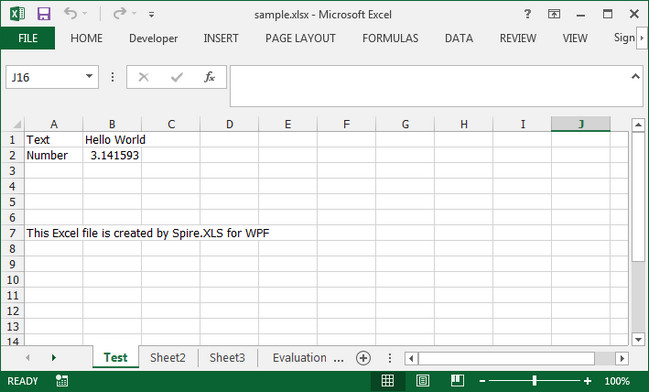# Create, Write and Save Excel File in WPF with C#, VB.NET

Creating, Writing and Saving Excel file are basic tasks in our daily life. This guide will demonstrate how to create an Excel file, insert some data and save the file with specified file format using Spire.XLS for WPF.

Apart from creating Excel from scratch, Spire.XLS also supports to load an existing Excel file, modify the data and do a large range of manipulations in Excel.

Code Snippets:

Step 1: Initialize a new instance of Workbook class. By default, three blank worksheets will be added into the workbook accordingly.

```Workbook workbook = new Workbook();
```

Step 2: Get the first worksheet from workbook and rename the sheet as "Test”.

```Worksheet sheet = workbook.Worksheets;
sheet.Name = "Test";
```

Step 3: Insert some text value and number value into the specified cells.

```sheet.Range["A1"].Text = "Text";
sheet.Range["A2"].Text = "Number";
sheet.Range["B1"].Text = "Hello World";
sheet.Range["B2"].NumberValue = 3.1415926;
sheet.Range["A7"].Text = "This Excel file is created by Spire.XLS for WPF";
```

Step 4: Save the file in the format of Excel 2013.

```workbook.SaveToFile("sample.xlsx",ExcelVersion.Version2013);
```

Output:Full Code:

[C#]
```using Spire.Xls;
using System.Windows;

namespace WpfApplication1
{
public partial class MainWindow : Window
{
public MainWindow()
{
InitializeComponent();
}
private void button1_Click(object sender, RoutedEventArgs e)
{
Workbook workbook = new Workbook();
Worksheet sheet = workbook.Worksheets;
sheet.Name = "Test";

sheet.Range["A1"].Text = "Text";
sheet.Range["A2"].Text = "Number";
sheet.Range["B1"].Text = "Hello World";
sheet.Range["B2"].NumberValue = 3.1415926;
sheet.Range["A7"].Text = "This Excel file is created by Spire.XLS for WPF";

workbook.SaveToFile("sample.xlsx", ExcelVersion.Version2013);
System.Diagnostics.Process.Start("sample.xlsx");

}
}
}
```
[VB.NET]
```Imports Spire.Xls
Imports System.Windows

Namespace WpfApplication1
Public Partial Class MainWindow
Inherits Window
Public Sub New()
InitializeComponent()
End Sub
Private Sub button1_Click(sender As Object, e As RoutedEventArgs)
Dim workbook As New Workbook()
Dim sheet As Worksheet = workbook.Worksheets(0)
sheet.Name = "Test"

sheet.Range("A1").Text = "Text"
sheet.Range("A2").Text = "Number"
sheet.Range("B1").Text = "Hello World"
sheet.Range("B2").NumberValue = 3.1415926
sheet.Range("A7").Text = "This Excel file is created by Spire.XLS for WPF"

workbook.SaveToFile("sample.xlsx", ExcelVersion.Version2013)
System.Diagnostics.Process.Start("sample.xlsx")

End Sub
End Class
End Namespace
```